Latest Banking jobs   »

# Quantitative Aptitude Quiz For IDBI AM/ Bank of India PO 2023-10th April

Direction (1-5): Find the approximate value of question marks (?) in following questions?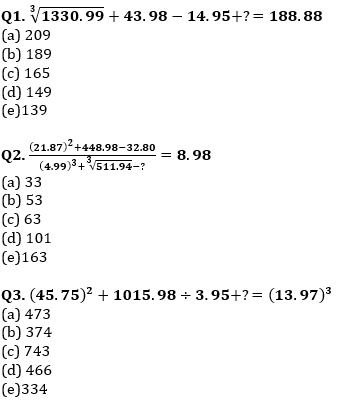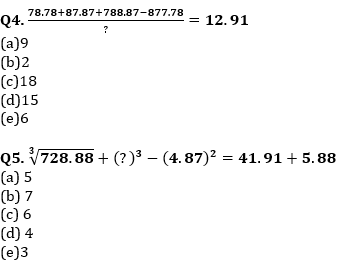Q6. In a mixture of milk and water, the proportion of milk by weight is 60%. If from the 80 gm mixture, 20 gm of mixture is taken out and 6 gm of pure water is added to the mixture then find the ratio of milk and water in the new mixture.
(a) 5 : 6
(b) 6 : 5
(c) 4 : 3
(d) 3 : 2
(e) 7 : 6

Q7. A sphere is melted and molded into solid cylinder. If radius of both solids is equal, then find the ratio of total surface area of sphere to the total surface area of cylinder
(a) 2 : 3
(b) 4 : 3
(c) 3 : 7
(d) 6 : 7
(e) 7 : 6

Q8. Three partners P, Q and R invested their amounts in ratio of 2 : 5 : 7. At the end of 6 months, ‘P’ added some more amount such that his investment become equals to half of sum of ‘Q’ and ‘R’ initial investment. If at the end of the year, Q’s share in profit is Rs 425, then find the total profit
(a) Rs 1250
(b) Rs 1360
(c) Rs 1840
(d) Rs 1050
(e)Rs 1450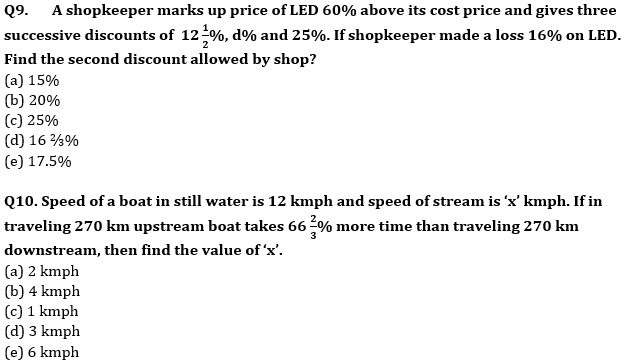Directions (11-15): Given bar graph shows no. of students (in thousands) who opted for three different specialization during the given five years in a university.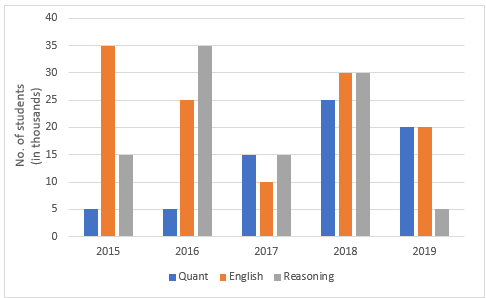Q11. Out of total number of students who opted for the given three subjects, in year 2019, 38% were girls. How many boys opted for reasoning in the same year?
(a) 1124
(b) 1536
(c) 1316
(d) Cannot be determined
(e) None of these

Q12. If the total number of students in the university in the year 2017 was 4,00,000, then the total number of students who opted for the given three subjects were what percent of the total students?
(a) 17%
(b) 10%
(c) 14%
(d) 7%
(e) 21%

Q13. What is the total number of students who opted for Quant and reasoning together in the years 2016, 2017 and 2019 together?
(a) 97000
(b) 93000
(c) 85000
(d) 96000
(e)95000

Q14. The total number of students who opted for Reasoning in the years 2015 and 2018 together are approximately what percent of the total number of students who opted for all three subjects in same years? (approx..)
(a) 36%
(b) 24%
(c) 44%
(d) 32%
(e) 46%

Q15. What is the respective ratio between the number of students who opted for English in the years 2016 and 2018 together to the number of students who opted for Quant in the years 2015 and 2019 together?
(a)11 : 5
(b)11 : 9
(c)11 : 7
(d)14 : 3
(e)13:7

Solutions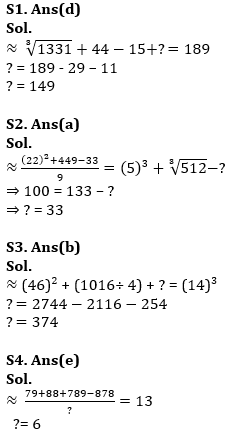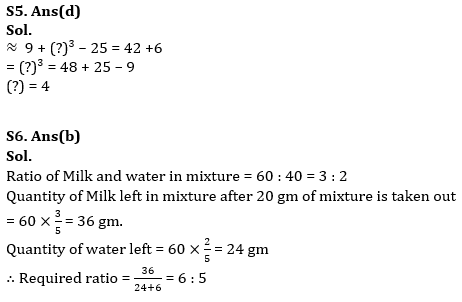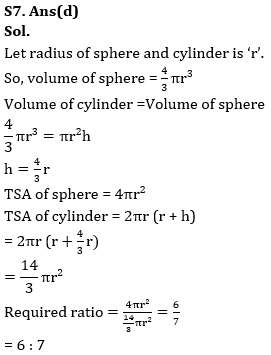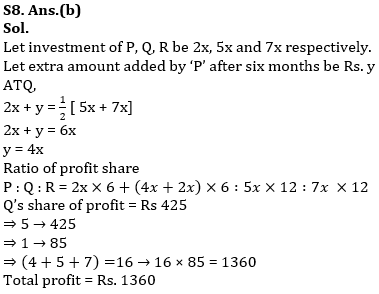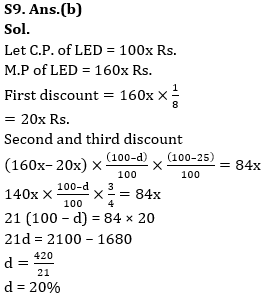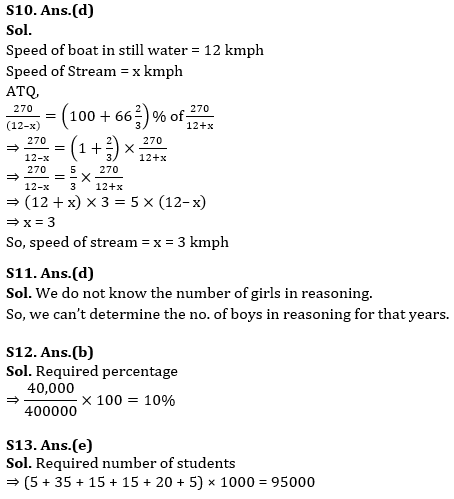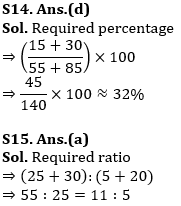## FAQs

### What is the selection process of the IDBI AM?

The selection process of IDBI AM is Online exam and Interview.

#### Congratulations!Union Budget 2023-24: Free PDF